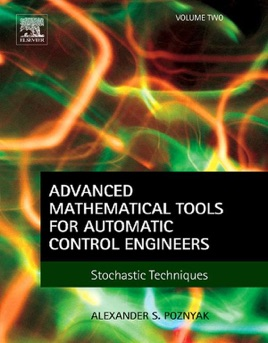• USD 229.99

## Descripción de editorial

Advanced Mathematical Tools for Automatic Control Engineers, Volume 2: Stochastic Techniques provides comprehensive discussions on statistical tools for control engineers.

The book is divided into four main parts. Part I discusses the fundamentals of probability theory, covering probability spaces, random variables, mathematical expectation, inequalities, and characteristic functions. Part II addresses discrete time processes, including the concepts of random sequences, martingales, and limit theorems. Part III covers continuous time stochastic processes, namely Markov processes, stochastic integrals, and stochastic differential equations. Part IV presents applications of stochastic techniques for dynamic models and filtering, prediction, and smoothing problems. It also discusses the stochastic approximation method and the robust stochastic maximum principle.
Provides comprehensive theory of matrices, real, complex and functional analysisProvides practical examples of modern optimization methods that can be effectively used in variety of real-world applicationsContains worked proofs of all theorems and propositions presented